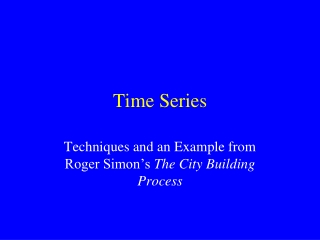DownloadDownload PresentationTime Series

# Time Series

Télécharger la présentation## Time Series

- - - - - - - - - - - - - - - - - - - - - - - - - - - E N D - - - - - - - - - - - - - - - - - - - - - - - - - - -
##### Presentation Transcript

1. Time Series Techniques and an Example from Roger Simon’s The City Building Process

2. What is a Time Series? • Numerical data ordered by intervals of time in chronological order • The analysis of a time series involves decomposing the series into its components, e.g., • Trends • Cyclical and Seasonal Fluctuations • Irregular Fluctuations

3. Example of a Time Series

4. To Analyze a Time Series… • Identify the possible processes affecting the series: • Inflation or Deflation (for series of prices or expenditures) • Long term trend • Cyclical fluctuations • Seasonal fluctuations • Irregular fluctuations

5. Steps to Analyze Changing Construction Costs • Identify a measure: • Construction Cost? Or…. • Construction Cost Per Square Foot • Convert to Constant Dollars • Measure the Trend • Identify cyclical or seasonal factors • Identify irregular fluctuations

6. Raw Construction Cost over Time

7. Properties Built over Time

8. Size of Buildings Built over Time

9. Adjusting for Inflation • if yrbuilt >887 and yrbuilt < 912 then let concost2=concost*20 • if yrbuilt >911 and yrbuilt < 916 then let concost2=concost/.06 • if yrbuilt = 916 then let concost2=concost/.065 • if yrbuilt = 917 then let concost2=concost/.077 • if yrbuilt = 918 then let concost2=concost/.090 • if yrbuilt = 919 then let concost2=concost/.104 • if yrbuilt = 920 then let concost2=concost/.120 • if yrbuilt = 921 then let concost2=concost/.107 • if yrbuilt = 922 then let concost2=concost/.103 • if yrbuilt = 923 then let concost2=concost/.102 • if yrbuilt = 924 then let concost2=concost/.102 • if yrbuilt = 925 then let concost2=concost/.105 • if yrbuilt = 926 then let concost2=concost/.106 • if yrbuilt = 927 then let concost2=concost/.104 • if yrbuilt = 928 then let concost2=concost/.102 • if yrbuilt = 929 then let concost2=concost/.102 • if yrbuilt=. then let concost2= concost *20 • if concost=. Then let concost2 =.

10. Construction Cost Adjusted for Inflation

11. Comparing Raw and Inflation Adjusted Construction Costs

12. Construction Cost per Square Foot (Inflation Adjusted)

13. Construction Cost per Square Foot (Inflation Adjusted)

14. The Model: Cost per square foot = constant(a) + b1*year + b2*(last year’s cost per square foot)

15. Cost per square foot = \$7.33 + .59 (last year’s cost) + .29 (year) Number of cases: 39 (1889-1928). 1889=1 Adjusted R Square: 77.2 The Model: Cost per square foot = constant(a) + b1*year + b2*last year’s cost per square foot

16. Trend and Residuals

17. The Data:1.Year 2. Cost Per Square Foot3. Last Year’s Cost Per Square Foot4. Predicted Cost5. Residual